# Open Organ Pipe

The pipe in which the both of its ends are open is called open organ pipe. Flutes is the example of organ pipe.

Different modes of vibration in open pipe: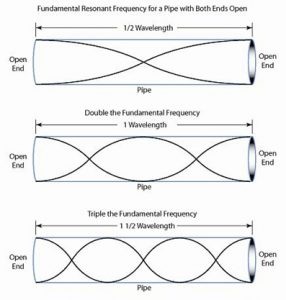1. First mode of vibration:
In the mode of vibration in the organ pipe, two antinodes are formed at two open ends and one node is formed in between them. If length of the pipe be ‘l’ and be the wavelength of wave emitted in this mode of vibration. Then,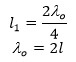If ‘μ’ be the velocity of sound and to be the frequency of wave in this mode of vibration. Then,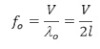This is the fundamental frequency of 1st overtone or 2 harmonious.

2. Second mode of vibration:
In the second mode of vibration in the open organ pipe, there antinodes are formed at two ends and two nodes between them.

If ‘l’ be the length of the pipe and λ1 be the wavelength of wave emitted in this mode of vibration. Then,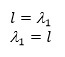If μ be the velocity of sound and to be the frequency of wave in this mode of vibration, then,This is the fundamental frequency of 2nd overtone or harmonics.

3. Third mode of vibration:
In the third mode of vibration in the open organ pipe, four antinodes are formed and three nodes between them. If ‘l’ be the length of the pipe and λ2 be the wavelength of wave emitted in this mode of vibration then,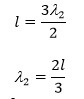If μ be the velocity of sound and f2 be the frequency of wavelength on this mode of vibration, then,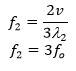This is the fundamental of 2nd overtone & 3rd harmonics.

In this way, for the 4th, 5th …………….. modes of vibration, the frequency of wave are f3 = 4f0; f4 = 5f0…………….are the frequency of 4th, 5th harmonics. Hence in open organ pipe odd and even both harmonic are present. Then the sound heard by open organ is so sonorous.